## Hypergeometric Series

A hypergeometric seriesis a series for which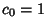and the ratio of consecutive terms is a Rational Function of the summation index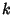, i.e., one for which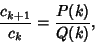(1)

withandPolynomials. The functions generated by hypergeometric series are called Hypergeometric Functions or, more generally, Generalized Hypergeometric Functions. If the polynomials are completely factored, the ratio of successive terms can be written(2)

where the factor of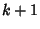in the Denominator is present for historical reasons of notation, and the resulting Generalized Hypergeometric Function is written(3)

Ifand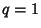, the function becomes a traditional Hypergeometric Function.

Many sums can be written as Generalized Hypergeometric Functions by inspections of the ratios of consecutive terms in the generating hypergeometric series.

Petkovsek, M.; Wilf, H. S.; and Zeilberger, D. Hypergeometric Series,'' How to Identify a Series as Hypergeometric,'' and Software That Identifies Hypergeometric Series.'' §3.2-3.4 in A=B. Wellesley, MA: A. K. Peters, pp. 34-42, 1996.